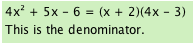### Home > CAAC > Chapter 12 > Lesson 12.1.3 > Problem12-25

12-25.
1. Add, subtract, multiply, or divide the following rational expressions. Simplify your answers, if possible. Homework Help ✎

1.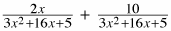2.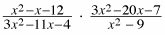3.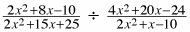4.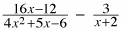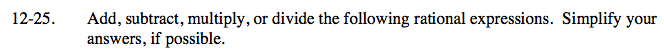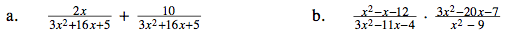Add the numerators and reduce. Factor and find any 1s.

Factor the expressions.
Reduce by looking for factors in the numerator and denominator that make 1.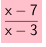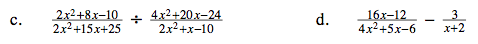Factor each expression. Look for 1s.